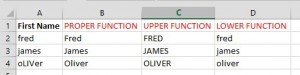# Changing text case in Excel

Excel does not have the same options as Word to change text case.

However, it doesn’t mean you can’t change text case it just means you use a different method to do it and this is by using functions.

PROPER          Will capitalise the first letter of each word
UPPER             Will change all letters to upper case
LOWER            Will change all letters to lower case

You will need to create new columns next to the columns of data you wish to change.  You can then delete the original columns afterwards.

In the example below I have created 3 new columns and used the three functions above to change the text case.The table below shows the cell references and then to the right of it the function used to produce the results above.

B2             =PROPER(A2)               B3               =PROPER(A3)           B4        =PROPER(A4)

C2             =UPPER(A2)                   C3               =UPPER(A3)              C4        =UPPER(A4)

D2             =LOWER(A2)                 D3              =LOWER(A3)             D4        =LOWER(A4)